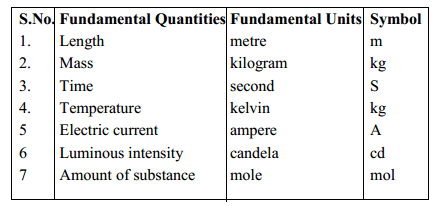# Cross Product

• Cross (vector) product of two vectors gives you a vector perpendicular to both whose magnitude is equal to the area of a parallelogram defined by the two initial vectors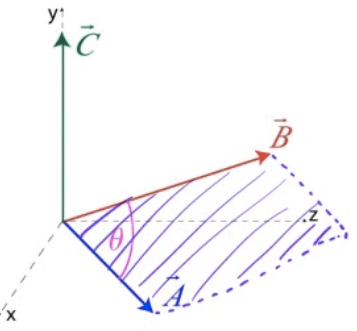• Positive direction of the cross product is given by the right-hand rule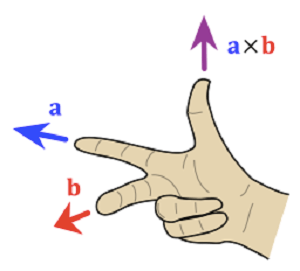• Cross product of parallel vectors is zero.

# Calculating the Cross Product

•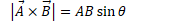•# Cross Product Properties

••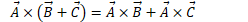•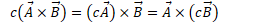•# Unites